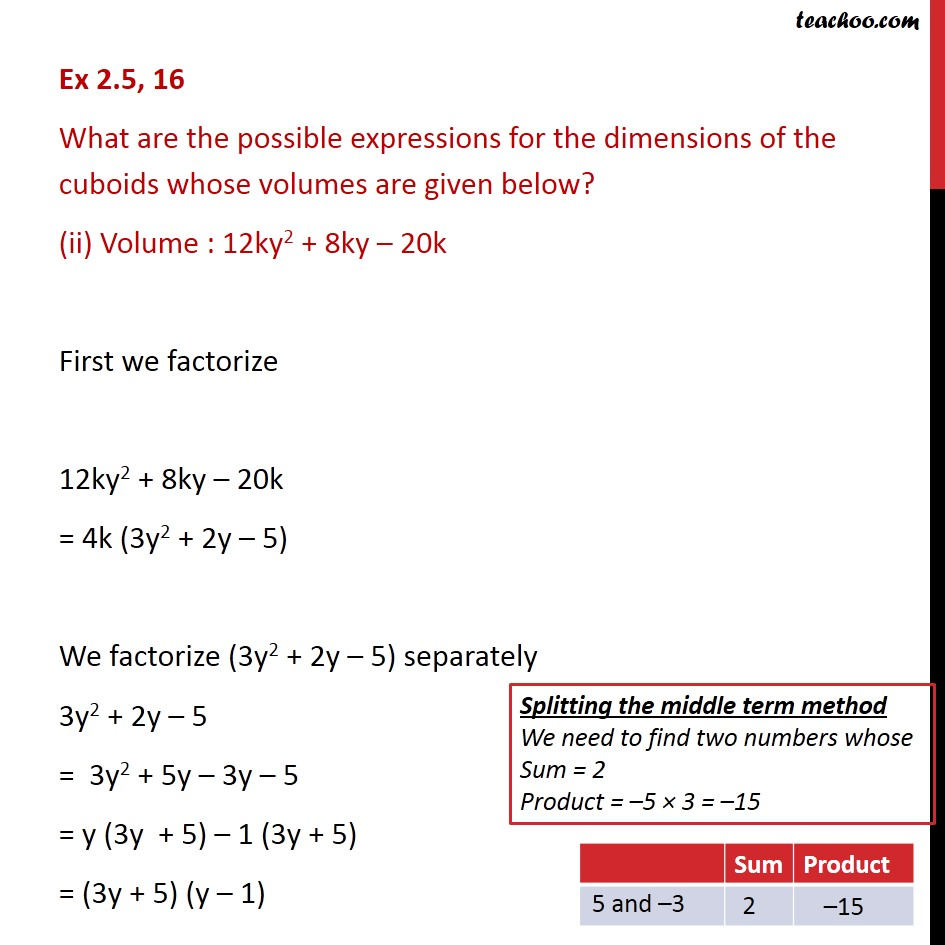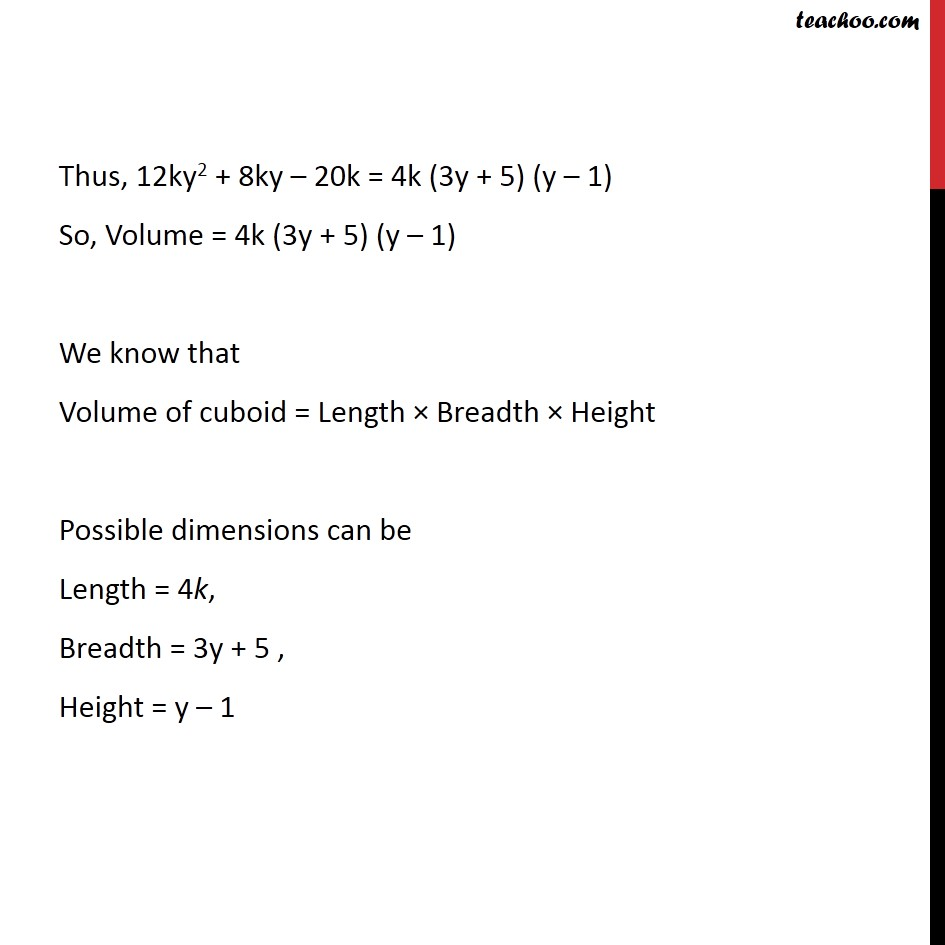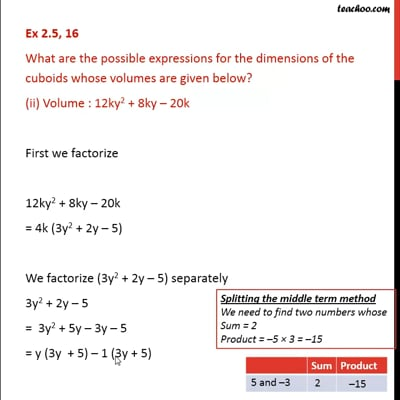Ex 2.5

Chapter 2 Class 9 Polynomials
Serial order wiseThis video is only available for Teachoo black users

Maths Crash Course - Live lectures + all videos + Real time Doubt solving!

### Transcript

Ex 2.5, 16 What are the possible expressions for the dimensions of the cuboids whose volumes are given below? (ii) Volume : 12ky2 + 8ky – 20k First we factorize 12ky2 + 8ky – 20k = 4k (3y2 + 2y – 5) We factorize (3y2 + 2y – 5) separately 3y2 + 2y – 5 = 3y2 + 5y – 3y – 5 = y (3y + 5) – 1 (3y + 5) = (3y + 5) (y – 1) Thus, 12ky2 + 8ky – 20k = 4k (3y + 5) (y – 1) So, Volume = 4k (3y + 5) (y – 1) We know that Volume of cuboid = Length × Breadth × Height Possible dimensions can be Length = 4k, Breadth = 3y + 5 , Height = y – 1